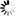DOI: 10.5176/2251-1911_CMCGS15.18

Authors: Baby Bhattacharya, Jayasree Chakraborty, and Arnab Paul

Abstract:  The Kernel of a set in a generalized topological space (GTS) is a recent concept which is published on 2013 in the Journal of Acta Mathematica Hungarica by Z. LI and W. ZHU. The aim of this paper is to introduce the same concept namely fuzzy Λ^(g_X ) -set and fuzzy V^(g_X )-set in the direction of generalized fuzzy topological spaces (GFTS). A few characterizations and properties are studied and investigated the topologies where these families of sets are involved. Particularly, our study is to show that most of the earlier defined different type of Λ-sets form an Alexanderoff space such as pre- Λ set and pre-V set, semi-pre Λ-set and semi pre-V set, Λ_m -set etc published in the above said journal in the year 2002, 2004, 2005 respectively, forms an Alexanderoff space in the ordinary topological space, but among the sets define in this paper only the family of V^(g_X )-sets form a generalized fuzzy topology and the family of Λ^(g_X ) -sets fails to form the same . Author introduced the concept of fuzzy contra (〖 g〗_X,g_Y) -continuity in generalized fuzzy topological spaces. In the present work the said notion is studied with the help of kernel of a set. The concept of fuzzy generalized Λ^(g_X )and fuzzy generalizedV^(g_X ) -sets are also studied.

Keywords: FuzzyΛ-closure operator, generalized fuzzy topological space (GFTS), fuzzy Λ^(g_X )-set, fuzzy V^(g_X ) -set, fuzzy generalized Λ^(g_X )-set, fuzzy generalized V^(g_X ) -set

simplr_role_lock:

Price: \$0.00Updating...